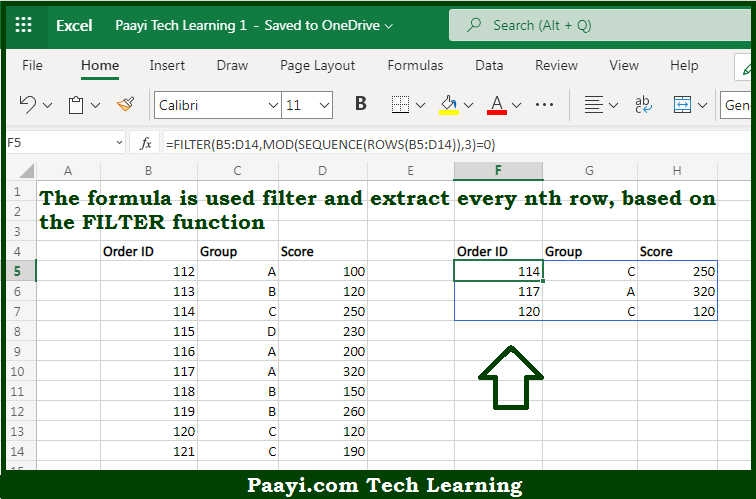# Learn How to Filter Every nth Row in Microsoft Excel

Written by | 0 Comments | 922 Views

In this article, you will learn how to evaluate things in Dynamic Arrays with formulas in Microsoft Excel using a single/combination(s) of functions. You will also know How to Filter Every nth Row and see the generic formula.

Learn How to Filter Every nth Row in Microsoft Excel

The main purpose of this formula is to filter and extract every nth row. Here we will learn how to filter every nth row in the given data range in the workbook in Microsoft Excel. That implies, with the help of a formula based on the FILTER, MOD, SEQUENCE, and ROW function you can able to filter and extract every nth row. So, with the help of this formula, you can able to filter every nth row in the given data range in the workbook in Microsoft Excel.

General Formula to Filter Every nth Row

=FILTER(rng,MOD (SEQUENCE(ROWS(rng)),n)=0)

The Explanation for the Filter Every nth RowSo we know that with the help of the given formula above you can able to filter and extract every nth row. Here we will learn how to filter every nth row in the given data range in the workbook in Microsoft Excel. As we know that the  FILTER function is utilized to filter and extract information based on logical criteria. The main goal of the example provided here is to extract every 3rd record from the data shown, but there is no row number information in the data. When we work from the inside out, the first step is to generate a set of row numbers. This is done with the SEQUENCE function.  So, with the help of this formula, you can able to filter and extract every nth row. Here we will learn how to filter every nth row in the given data range in the workbook in Microsoft Excel.